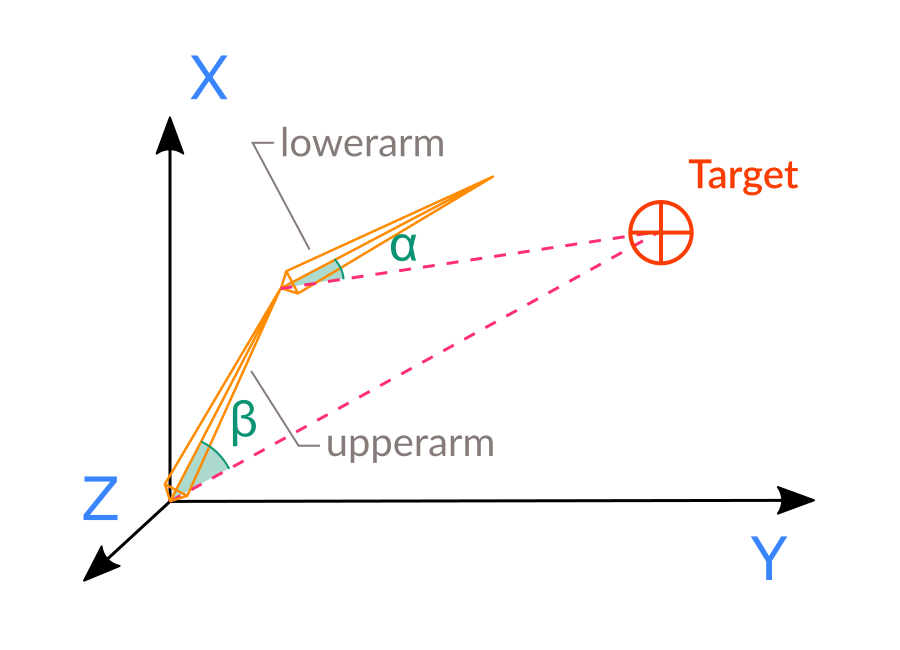Inverse kinematics¶

This tutorial is a follow-up of Working with 3D skeletons.

Before continuing on, I’d recommend reading some theory, the simplest article I find is this:

http://freespace.virgin.net/hugo.elias/models/m_ik2.htm

Initial problem¶

Talking in Godot terminology, the task we want to solve here is position our 2 angles we talked about above so, that the tip of lowerarm bone is as close to target point, which is set by target Vector3() as possible using only rotations. This task is very calculation-intensive and never resolved by analytical equation solve. So, it is an underconstrained problem, which means there is unlimited number of solutions to the equation.For easy calculation, for this chapter we consider target is also child of Skeleton. If it is not the case for your setup you can always reparent it in your script, as you will save on calculations if you do.

In the picture you see angles alpha and beta. In this case we don’t use poles and constraints, so we need to add our own. On the picture the angles are 2D angles living in plane which is defined by bone base, bone tip and target.

The rotation axis is easily calculated using cross-product of bone vector and target vector. The rotation in this case will be always in positive direction. If t is the Transform which we get from get_bone_global_pose() function, the bone vector is

t.basis

So we have all information here to execute our algorithm.

In game dev it is common to resolve this problem by iteratively closing to the desired location, adding/subtracting small numbers to the angles until the distance change achieved is less than some small error value. Sounds easy enough, but there are Godot problems we need to resolve there to achieve our goal.

• How to find coordinates of the tip of the bone?
• How to find vector from bone base to target?

For our goal (tip of the bone moved within area of target), we need to know where the tip of our IK bone is. As we don’t use a leaf bone as IK bone, we know the coordinate of the bone base is the tip of parent bone. All these calculations are quite dependent on the skeleton’s structure. You can use pre-calculated constants as well. You can add an extra bone for the tip of IK and calculate using that.

Implementation¶

We will just use exported variable for bone length to be easy.

export var IK_bone="lowerarm"
export var IK_bone_length=1.0
export var IK_error = 0.1

Now, we need to apply our transformations from IK bone to the base of chain. So we apply rotation to IK bone then move from our IK bone up to its parent, then apply rotation again, then move to the parent of current bone again, etc. So we need to limit our chain somewhat.

export var IK_limit = 2

var skel
skel = get_node("arm/Armature/Skeleton")
set_process(true)

Now we can write our chain-passing function:

func pass_chain():
var b = skel.find_bone(IK_bone)
var l = IK_limit
while b >= 0 and l > 0:
print( "name":", skel.get_bone_name(b))
print( "local transform":", skel.get_bone_pose(b))
print( "global transform":", skel.get_bone_global_pose(b))
b = skel.get_bone_parent(b)
l = l - 1

And for the _process() function:

func _process(dt):
pass_chain(dt)

Executing this script will just pass through bone chain printing bone transforms.

extends Spatial

export var IK_bone="lowerarm"
export var IK_bone_length=1.0
export var IK_error = 0.1
export var IK_limit = 2
var skel
skel = get_node("arm/Armature/Skeleton")
set_process(true)
func pass_chain(dt):
var b = skel.find_bone(IK_bone)
var l = IK_limit
while b >= 0 and l > 0:
print("name: ", skel.get_bone_name(b))
print("local transform: ", skel.get_bone_pose(b))
print( "global transform:", skel.get_bone_global_pose(b))
b = skel.get_bone_parent(b)
l = l - 1
func _process(dt):
pass_chain(dt)

Now we need to actually work with target. The target should be placed somewhere accessible. Since “arm” is imported scene, we better place target node within our top level scene. But for us to work with target easily its Transform should be on the same level as Skeleton.

To cope with this problem we create “target” node under our scene root node and at script run we will reparent it copying global transform, which will achieve wanted effect.

Create new Spatial node under root node and rename it to “target”. Then modify _ready() function to look like this:

var skel
var target